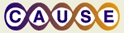# Nature of the chi-square distributionThis material was originally developed through CAUSE
as part of its collaboration with the SERC Pedagogic Service.

#### Summary

In this activity, students learn the true nature of the chi-square and F distributions in lecture notes (PowerPoint file) and an Excel simulation. This leads to a discussion of the properties of the two distributions. Once the sum of squares aspect is understood, it is only a short logical step to explain why a sample variance has a chi-square distribution and a ratio of two variances has an F-distribution.

In a subsequent activity, instances of when the chi-square and F-distributions are related to the normal or t-distributions (e.g. Chi-square = z2, F = t2) will be illustrated. Finally, the activity will conclude with a brief overview of important applications of chi-square and F distributions, such as goodness-of-fit tests and analysis of variance.

link text (Microsoft Word 3.5MB May17 07)

## Learning Goals

In this first activity students will learn the important lesson that statistical distributions, such as the normal, Student's t, chi-square and F distributions are interrelated.

In the second activity, they will be encouraged to explore these relationships and to discover equivalent statistical tests that can used in specific situations. The relationship between the chi-square and z distributions will be underscored by demonstrating that when testing for the equality of two population proportions by two different methods a computed chi-square value will, in fact, be the square of the normal distribution z-value for the corresponding test.

Likewise, in simple linear regression a test of hypothesis for the slope of a regression line can be performed using t-test or F-tests, where the computed F-value is the square of the corresponding t-value.

## Context for Use

The activity can be undertaken at different levels and with different degrees of rigor. At the simplest level it is used to introduce the chi-square distribution as the sampling distribution of a sample variance. This is necessary for inferences concerning a population variance (confidence interval, test of hypothesis). This activity consists of using software (Excel, Minitab, Fathom,...) to generate random samples of a normal variate and then to show that the resulting sum of X and sum of X2 approximate a Student's t-distribution and chi-square distribution, respectively.

The simplest activity, as described above, will work well in the large class as a demonstration, or in a computer lab as a hands-on activity, and can be accomplished in one class period.

The second activity introduces more sophisticated connections involving the chi-square and the F-distributions, and shows how these can be demonstrated through goodness-of-fit tests, ANOVA and regression analyses. These more advanced procedures are appropriate in the latter part of the introductory course or early in the second course when discussing tests of hypothesis for population variances, goodness-of-fit tests, and regression analysis.

## Description and Teaching Materials

• The activity can be introduced in lecture format with the Powerpoint File describing the chi-square distribution as the sum of squares of values selected from a normal distribution, and showing the relationship with the Student t-distribution represented by the sum of the same 10 values.
• A simulation can then be demonstrated using the Excel file containing 2,000 samples (rows) of data in which each sample contains 10 randomly generated values from a standard normal distribution. Each row also shows the sum of the ten values and the sum of the squares of the 10 values. Histograms are drawn for both the sum and the sum of squares. Pressing the F9 (recalculate) key in Excel causes the entire spreadsheet to be recalculated and the histograms to be redrawn. It is evident from the simulation that the sum of the 10 values generates an almost symmetrical distribution, approximated by Student's t-distribution, while the sum of squares of the 10 values generates a positively skewed histogram, consistent with the chi-square distribution.
• A second Excel file includes three worksheets demonstrating (1) the relationship between Chi-Square and z distribution in a test for equal proportions and (2) the relationship between F and t distributions in ANOVA and regression examples
link text (Excel 918kB May16 07) link text (PowerPoint 274kB May17 07) link text (Excel 24kB May17 07)

## Teaching Notes and Tips

This first activity will normally be introduced as an introduction to inferences concerning a population variance. Students will already be familiar with the idea that the mean (and therefore the sum) of values from a normally distributed variable will follow a specific distribution, namely Student's t. It is natural to ask whether the sum of squares of these same values also has a particular distribution. This is where the chi-square distribution fits into the course. Once this concept is established, it is easy to demonstrate that the variance is simply a sum of squares multiplied by a constant (the degrees of freedom) and therefore it will follow a chi-square distribution.

The activity can be introduced in a single class period of at least 50 minutes duration.

An effective way to present the activity is to show the PowerPoint presentation (file chisquare.ppt) followed by the Excel simulation (file ChiSquareSimulation.xls). The instructor wil then be ready to move on to a discussion of the use of the chi-square tables in interval estimation and hypothesis testing examples.

## Assessment

Assessment primarily by devising short test questions to determine student understanding of concepts.
• Display histograms and ask students to identify underlying distribution.
• Ask students to match distribution graphs to types of hypothesis test.
• Match sample statistic (mean, total, proportion, variance...) to associated distribution.

## References and Resources

Links to various applets - still to be developed. The links supplement the ideas developed here regarding the properties of the chi-square distribution, but they do not explicitly discuss chi-square in terms of the ideas developed in this activity, namely as a sum of squares.